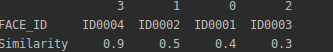x = np.array([('ID0001', 0.4), ('ID0002', 0.5), ('ID0003', 0.3), ('ID0004', 0.9)],dtype=[('FACE_ID', 'U10'), ('Similarity', 'f8')])

y = pd.DataFrame(x).sort_values('Similarity', ascending=False).TID0004 0.9 ID0002 0.5 ID0001 0.4 ID0003 0.3

1个回答

``````result = zip(df.iloc[::2], df.iloc[1::2])
print(result)
``````